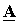# Phase Coordinates Method: Short Curcuit Model

In the phase coordinate method for modeling damage in the power line, the corresponding damage model is used with its transmission matrix. The damage model is composed of a set of active resistances.

In fig. 1 shows a fault model for short circuits. The model allows simulating symmetric three-phase K(3) and asymmetric single-phase K(1), interphase K(2) and two-phase K(1,1) earth faults. In this case, the active resistances vary in the range from 0 to ∞.Fig. 1. Power line fault model

In the table 1 shows the resistance variations for different types of damage.

Tab. 1. Resistance values for different types of short circuits

 Type RA RB RC RAB RBC RCA KA(1) RA ∞ ∞ ∞ ∞ ∞ KB(1) ∞ RB ∞ ∞ ∞ ∞ KC(1) ∞ ∞ RC ∞ ∞ ∞ KAB(2) ∞ ∞ ∞ RAB ∞ ∞ KBC(2) ∞ ∞ ∞ ∞ RBC ∞ KCA(2) ∞ ∞ ∞ ∞ ∞ RCA KAB(1,1) RA RB ∞ RAB ∞ ∞ KBC(1,1) ∞ RB RC ∞ RBC ∞ KCA(1,1) RA ∞ RC ∞ ∞ RCA K(3) RA RB RC ∞ ∞ ∞

The direct transmission matrixis determined by the expression,

where,,,,,,,,– resistances of the damage model (transient resistances at the point of short circuit).

The attached file contains the calculation of the matrixof the damage model form in Matlab: A_fault.m

This site uses Akismet to reduce spam. Learn how your comment data is processed.